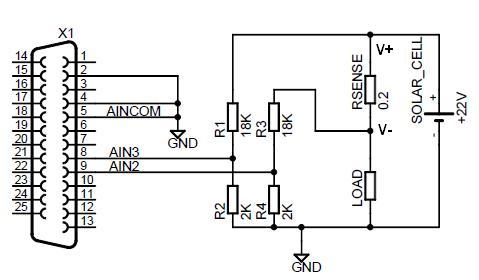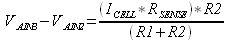The following information is for the EMANT300, EMANT380

# Measure Power of a Solar Cell

Question: I have a 6 to 10W solar panel and would like to monitor its output voltage and current. The Voc is 22V and its short circuit current is 0.6A.

WARNING: Note that the max input voltage with respect to GND for the EMANT380 Bluetooth DAQ is 3.3V. For the EMANT300 USB DAQ, the max input voltage with respect to GND is 5V. The min input voltage is the same for both at 0V.

To measure the cell voltage of 22V, we will need to attenuate the voltage to meet the input requirements of the EMANT3X0 modules. For the current measurement, we measure the voltage drop across a sense resistor. A possible schematic is shown belowThe voltage at AIN3 is attenuated by 10 for the resistor values given. The voltage equation isBy reading the voltage between AIN3 and AINCOM and multiplying by the attenuating factor of 10, we obtain the solar cell voltage.

The current of the solar cell ICELL is measured using the sense resistor RSENSE. Using a 0.2 ohm sense resistor, the voltage drop across RSENSE at 0.6A is 120mV. In this instance a 0.25W resistor is sufficient.

Applying the same formula to VAIN2 and since R1 = R3 and R2 = R4 and simplifying, we obtain the relation between the measured voltage and the solar cell current.Note:

1. The voltage at the inputs should not be less than 0 or greater than 5V (for EMANT300) or 3.3V (for the EMANT380) to avoid damage to the inputs. See Analog Input Range specification with Buffer On and Buffer Off
2. If the solar cell ground and EMANT300 ground are at the different potentials, you may damage the EMANT300. Connecting the grounds together will create a ground loop.
3. For the EMANT380, if you power it by a battery or from the solar cell, this ground problem is avoided. The EMANT380 requires a 3.3V supply.
4. R2 = R4 should not be less than 2K to avoid loading.
5. Don't forget to multiply the measured voltage with the inverse of the attenuation factor
6. The selection of the RSENSE value depends on how much voltage drop you can accept in series with the load and the resolution of the measured current.
7. Calculate the power rating of RSENSE.
8. Use 1% tolerance or better resistors. We have not considered the tolerance in our calculations. One quick way to account for the error is to short RSENSE, measure the error voltage and subtract this value from subsequent measurements.